• +91 9971497814
• info@interviewmaterial.com

# RD Chapter 12- Heron-s Formula Ex-12.3 Interview Questions Answers

### Related Subjects

Question 1 : In two right triangles one side an acute angle of one are equal to the corresponding side and angle of the other. Prove that the triangles are congruent.

Given : In ∆ABC and ∆DEF,
∠B = ∠E = 90°
∠C = ∠F
AB = DE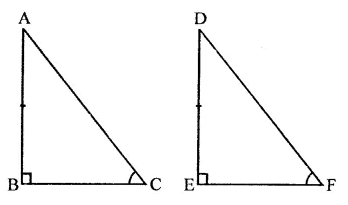To prove : ∆ABC = ∆DEF
Proof : In ∆ABC and ∆DEF,
∠B = ∠E (Each = 90°)
∠C = ∠F (Given)
AB = DE (Given)
∆ABC = ∆DEF (AAS axiom)

Question 2 : If the bisector of the exterior vertical angle of a triangle be parallel to the base. Show that the triangle is isosceles.

Given : In ∆ABC, AE is the bisector of vertical exterior ∠A and AE ∥ BC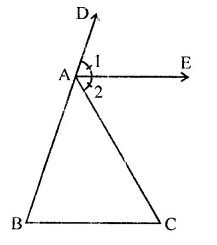To prove : ∆ABC is an isosceles
Proof: ∵ AE ∥ BC
∴ ∠1 = ∠B (Corresponding angles)
∠2 = ∠C (Alternate angle)
But ∠1 = ∠2 (∵ AE is the bisector of ∠CAD)
∴ ∠B = ∠C
∴ AB = AC (Sides opposite to equal angles)
∴ ∆ABC is an isosceles triangle

Question 3 : In an isosceles triangle, if the vertex angle is twice the sum of the base angles, calculate the angles of the triangle.

Given : In ∆ABC, AB = AC
∠A = 2(∠B + ∠C)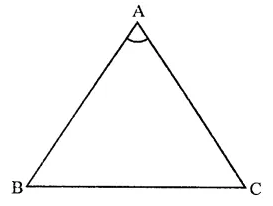To calculate: Base angles,
Let ∠B = ∠C = x
Then ∠A = 2(∠B + ∠C)
= 2(x + x) = 2 x 2x = 4x
∵ Sum of angles of a triangle = 180°
∴ 4x + x + x – 180° ⇒ 6x = 180°
⇒ x=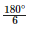= 30° o
∴ ∠B = ∠C = 30 and ∠A = 4 x 30° = 120

Question 4 : Prove that each angle of an equilateral triangle is 60°. [NCERT]

Given : ∆ABC is an equilateral triangle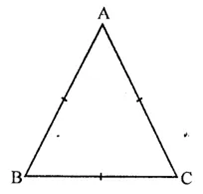Proof: In ∆ABC,
AB = AC (Sides of an equilateral triangle)
∴ ∠C = ∠B …(i)
(Angles opposite to equal angles)
Similarly, AB = BC
∴ ∠C = ∠A …(ii)
From (i) and (ii),
∠A = ∠B = ∠C
But ∠A + ∠B + ∠C = 180° (Sum of angles of a triangle)
∴ ∠A = ∠B = ∠C =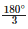= 60°

Question 5 : Angles A, B, C of a triangle ABC are equal to each other. Prove that ∆ABC is equilateral.

Given : In ∆ABC, ∠A = ∠B = ∠C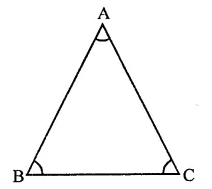To prove : ∆ABC is an equilateral
Proof: In ∆ABC,
∴ ∠B = ∠C (Given)
∴ AC = AB …(i) (Sides opposite to equal angles)
Similarly, ∠C = ∠A
∴ BC =AB …(ii)
From (i) and (ii)
AB = BC = CA
Hence ∆ABC is an equilateral triangle

Question 6 : ABC is a right angled triangle in which ∠A = 90° and AB = AC. Find ∠B and ∠C.

In ∆ABC, ∠A = 90°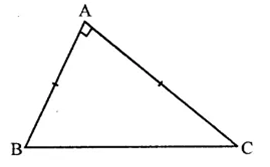AB =AC (Given)
∴ ∠C = ∠B (Angles opposite to equal sides)
But ∠B + ∠C = 90° (∵ ∠B = 90°)
∴ ∠B = ∠C =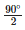= 45°
Hence ∠B = ∠C = 45°

Question 7 : PQR is a triangle in which PQ = PR and S is any point on the side PQ. Through S, a line is drawn parallel to QR and intersecting PR at T. Prove that PS = PT.

Given : In ∆PQR, PQ = PR
S is a point on PQ and PT || QR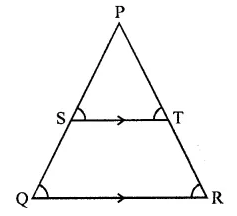To prove : PS = PT
Proof : ∵ST || QR
∴ ∠S = ∠Q and ∠T = ∠R (Corresponding angles)
But ∠Q = ∠R (∵ PQ = PR)
∴ PS = PT (Sides opposite to equal angles)

Question 8 : In a ∆ABC, it is given that AB = AC and the bisectors of ∠B and ∠C intersect at O. If M is a point on BO produced, prove that ∠MOC = ∠ABC.

Given : In ∆ABC, AB = AC the bisectors of ∠B and ∠C intersect at O. M is any point on BO produced.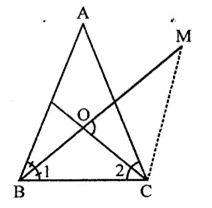To prove : ∠MOC = ∠ABC
Proof: In ∆ABC, AB = BC
∴ ∠C = ∠B
∵ OB and OC are the bisectors of ∠B and ∠C
∴ ∠1 =∠2 =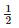∠B
Now in ∠OBC,
Ext. ∠MOC = Interior opposite angles ∠1 + ∠2
= ∠1 + ∠1 = 2∠1 = ∠B
Hence ∠MOC = ∠ABC

Question 9 : P is a point on the bisector of an angle ∠ABC. If the line through P parallel to AB meets BC at Q, prove that triangle BPQ is isosceles.

Given : In ∆ABC, P is a point on the bisector of ∠B and from P, RPQ || AB is draw which meets BC in Q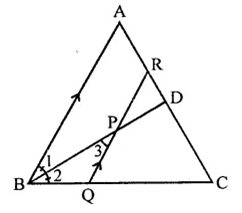To prove : ∆BPQ is an isosceles
Proof : ∵ BD is the bisectors of CB
∴ ∠1 = ∠2
∵ RPQ || AB
∴ ∠1 = ∠3 (Alternate angles)
But ∠1 == ∠2 (Proved)
∴ ∠2 = ∠3
∴ PQ = BQ (sides opposite to equal angles)
∴ ∆BPQ is an isosceles

Question 10 :  ABC is a triangle in which ∠B = 2∠C, D is a point on BC such that AD bisects ∠BAC = 72°.

Given: In ∆ABC,
∠B = 2∠C, AD is the bisector of ∠BAC AB = CD
To prove : ∠BAC = 72°
Construction : Draw bisector of ∠B which meets AD at O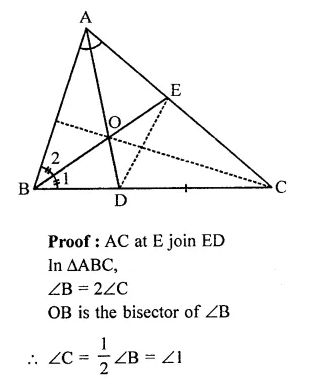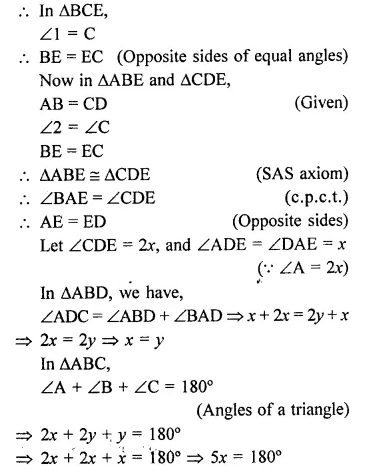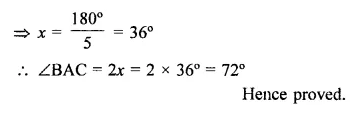Todays Deals### RD Chapter 12- Heron-s Formula Ex-12.3 Contributorskrishan

Name:
Email:

# Latest News# 9000 interview questions in different categories